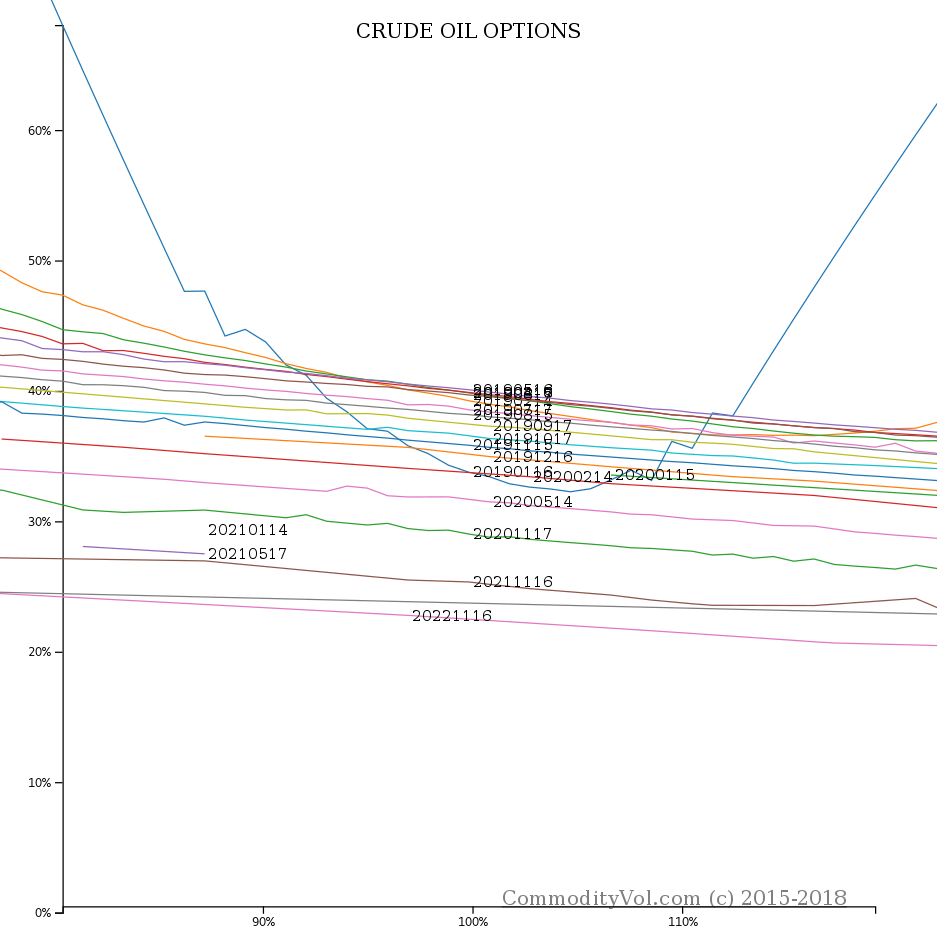### NYMEX LO Jan 11, 2019#### At the money vols and strikes (approx)

• LO F 2019 Vol= 0.3376 Strike= 51.5
• LO G 2019 Vol= 0.3897 Strike= 52
• LO H 2019 Vol= 0.3943 Strike= 52.5
• LO J 2019 Vol= 0.3955 Strike= 52.5
• LO K 2019 Vol= 0.3956 Strike= 53
• LO M 2019 Vol= 0.3912 Strike= 53.5
• LO N 2019 Vol= 0.3797 Strike= 53.5
• LO Q 2019 Vol= 0.3752 Strike= 54
• LO U 2019 Vol= 0.3655 Strike= 55
• LO V 2019 Vol= 0.3587 Strike= 54
• LO X 2019 Vol= 0.3515 Strike= 54
• LO Z 2019 Vol= 0.3418 Strike= 55
• LO F 2020 Vol= 0.3354 Strike= 55
• LO G 2020 Vol= 0.3288 Strike= 55
• LO H 2020 Vol= 0.2821 Strike= 75
• LO J 2020 Vol= 0.2751 Strike= 75
• LO K 2020 Vol= 0.3109 Strike= 53.5
• LO M 2020 Vol= 0.2643 Strike= 75
• LO N 2020 Vol= 0.2609 Strike= 75
• LO Q 2020 Vol= 0.2576 Strike= 75
• LO U 2020 Vol= 0.2546 Strike= 75
• LO V 2020 Vol= 0.2522 Strike= 75
• LO X 2020 Vol= 0.2836 Strike= 54
• LO F 2021 Vol= 0.2933 Strike= 45
• LO K 2021 Vol= 0.2750 Strike= 45
• LO X 2021 Vol= 0.2483 Strike= 53
• LO X 2022 Vol= 0.2160 Strike= 56
• LO X 2023 Vol= 0.2524 Strike= 30.5
The information above is the product code, expiration month codethe exact last day of trading is in the brackets finally we have the expiry year. This is generally not the standard form for symbology. However, with some contracts we may have two or more expiries with the same contract month. The contracts may even share expiry year, so in order to disambiguate the contracts we show extra detail.The vol is next, and finally the actual strike.